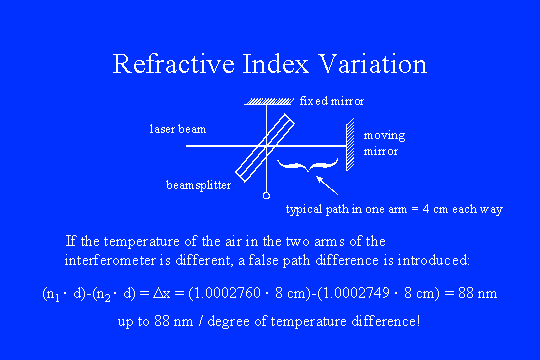Back ] Up ] Next ]

Sensitivity Issues in Step-Scan FT-IR SpectrometryThis is the most important slide in the entire sequence. The optical path of the infrared and laser beams through the interferometer is the product of the physical distance and the optical index. If the temperature varies between the two arms, a false path difference can be introduced. The refractive index of air at 15 °C is 1.0002760, while at 16 °C is it is 1.0002749 (a difference of about 1 ppm). A typical two-way path in each of the arms is 8 cm, so up to 88 nm of error, per degree of temperature difference, can be introduced. If the difference were stable or homogeneous it would not be so serious. However, temperature differences are induced by convective heat transfer and are neither stable nor homogeneous. To obtain a SNR of 10,000:1 using interferometric measurements in the mid-IR, the RMS error in sampling position must be held to less than 1 nm. Hence, the effective temperature difference between the two arms of the interferometer should be less than 1/100 of a degree. Now we can look for experimental verification of these ideas.